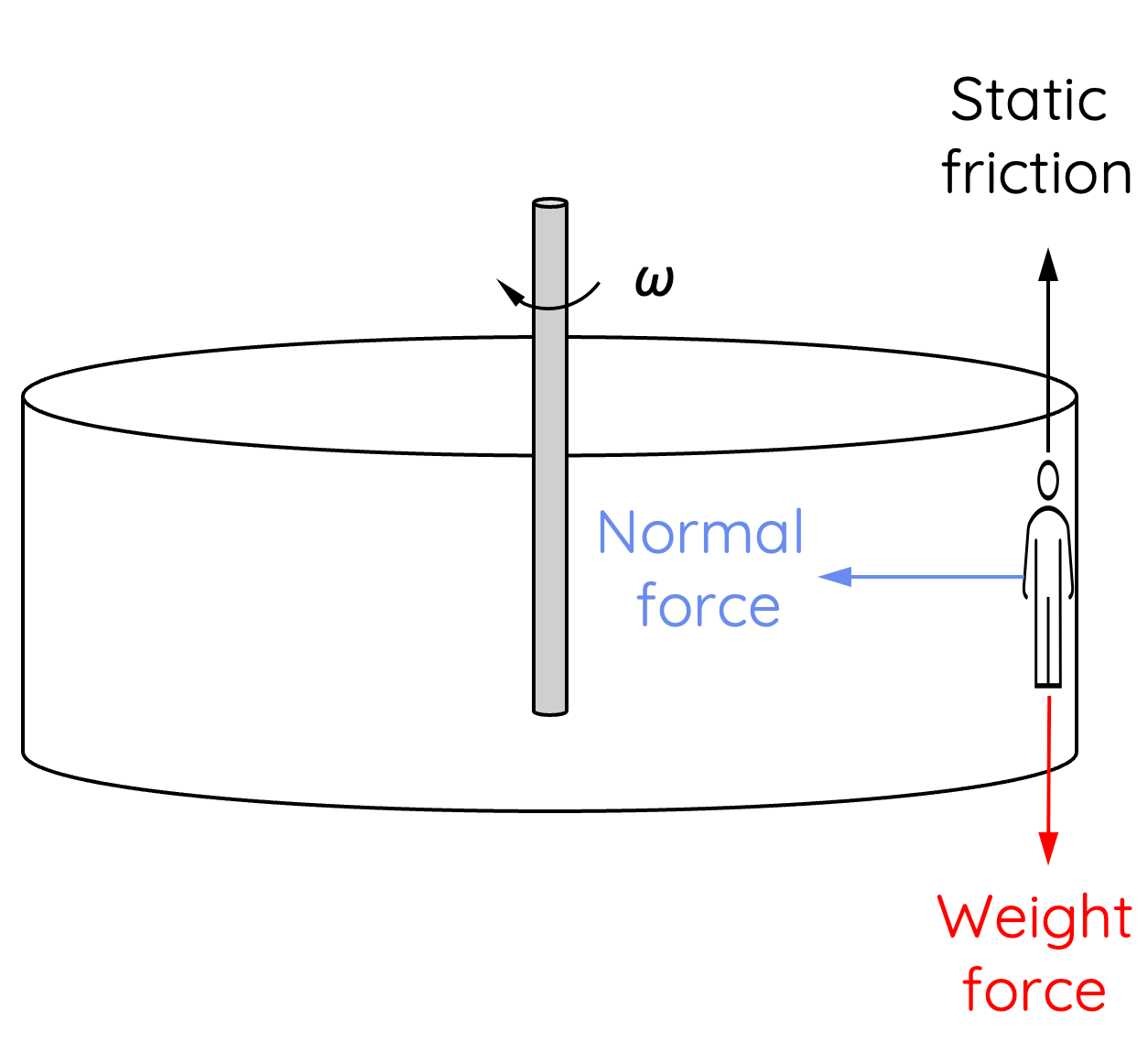# Circular Motion Involving Normal Force

This topic is part of the HSC Physics course under the section Circular Motion.

### HSC Physics Syllabus

• analyse the forces acting on an object executing uniform circular motion in a variety of situations, for example:

- cars moving around horizontal circular bends
- a mass on a string
- objects on banked tracks

### Circular Motion Involving Normal Forces

For HSC Physics students, it's essential to grasp the concept of circular motion in various scenarios. This article will illuminate the dynamic interplay of forces in this context, with a special focus on the normal force.

### Horizontal Circular Motion Involving Normal Force

A classic example of circular motion involving normal force is the 'rotor' ride.In the rotor, riders stand with their back against the wall. As the rotor spins, each person experiences an outward centrifugal force, causing them to be pressed against the wall. The wall then exerts a normal force on the person towards the centre of the circular motion.In this instance, the centripetal force is provided by the normal force, such that:

$$N = \frac{mv^2}{r}$$

As the rotational (angular) speed increases, the magnitude of normal force increases.

The wall also exerts an upward static friction force, which is given by:

$$f_{\text{static}} = \mu N$$

As the magnitude of normal force increases, the magnitude of static friction also increases until it balances the downward weight force of the person. When this occurs, the net force in the vertical axis acting on the person is zero.

$$f_{\text{static}} = mg$$

$$\mu N = mg$$

$$\mu \times \frac{mv^2}{r} = mg$$

$$\frac{\mu v^2}{r} = g$$

Rearranging the equation, we can derive an expression for velocity of the rotor required to suspend the person on the wall:

$$v = \sqrt{\frac{gr}{\mu}}$$

This equation tells us that the required velocity is independent of the person's mass. It is dependent on:

• Radius of the rotor: greater the radius, greater the velocity needed.
• Acceleration due to gravity
• Coefficient of static friction: greater the coefficient of friction, smaller the velocity needed.

### Setting the Scene: Vertical Semicircular Paths

Imagine a roller coaster car or a small ball moving inside a vertically oriented loop. As it travels the path, it encounters both the highest and lowest points of the semicircle. Here, the role of the normal force is vital, changing in magnitude and even direction based on the object's position.

### Force at the Top of the Loop

At the top of the loop, both the weight force of the object and the normal force the surface exerts on the objects are directed downward.

Since the direction of centripetal force is directed downward, it is given by the sum of weight and normal force:

$$F_{\text{centripetal}} = mg + N$$

$$N = F_{\text{centripetal}} - mg$$

### Force at the Bottom of the Loop

At the bottom of the loop, the weight force acts downward, and the normal force the surface exerts on the object acts upward.

Since the direction of the centripetal force at the bottom of the loop is directed upward, it is given by:

$$F_{\text{centripetal}} = N - mg$$

$$N = F_{\text{centripetal}} + mg$$

If the object is to undergo uniform circular motion, that is with a constant centripetal force, the magnitude of normal force would be greater at the bottom of the loop compared to the top of the loop.

### Example

A 2000 kg car travels on a road at a speed of 20 m s–1. The radius of the curvature at two points A and B are both 50 m.(a) Calculate the normal force exerted by the road at points A and B.

(b) Calculate the maximum speed that the car can travel at point B without losing contact with the road.

Solution for part (a):

At point A, the centripetal force is directed upward so it is given by:

$$F_{\text{centripetal}} = N - mg$$

$$N = \frac{mv^2}{r} + mg$$

$$N = \frac{(2000)(20)^2}{50} + (2000)(9.8) = 3.56 \times 10^4 \, \text{N upward}$$

At point B, the centripetal forward is directed downward so it is given by:

$$F_{\text{centripetal}} = mg - N$$

$$N = mg - \frac{mv^2}{r}$$

$$N = (2000)(9.8) - \frac{(2000)(20)^2}{50} = 3600 \, \text{N upward}$$

Solution for part (b):

As the car's speed increases, the magnitude of normal force decreases. This should make sense as a greater speed causes the car to lose contact with the ground. Therefore, maximum speed occurs when the normal force is zero.

$$\frac{mv^2}{r} = mg$$

$$\frac{v^2}{r} = g$$

$$v = \sqrt{rg} = \sqrt{(50)(9.8)}$$

$$v = 22.1 \, m \, s^{-1}$$